# RD Sharma Solutions Class 8 Understanding Shapes Polygons Exercise 15.1

## RD Sharma Solutions Class 8 Chapter 15 Exercise 15.1

#### Exercise 15.1

1. Draw rough diagrams to illustrate the following:

(i) Open curve

(ii) Closed curve

Solution:

(i) Open curve: A curve in which the beginning and the end points does not cut each other or are different.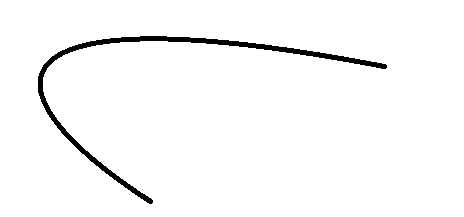(ii) Closed curve: A curve in which the beginning and the end points are the same and cuts each other.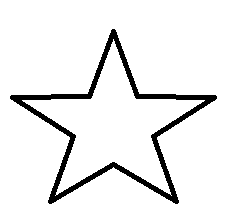2. Classify the following curves as open or closed: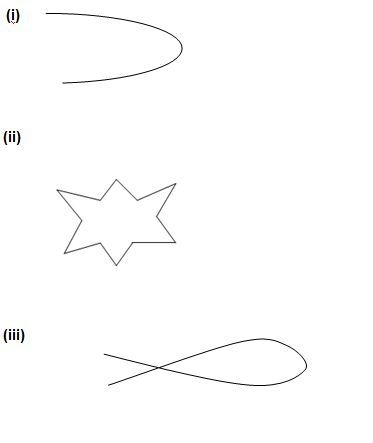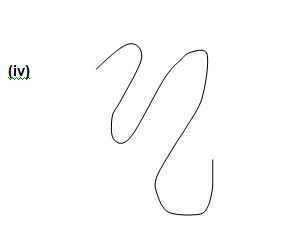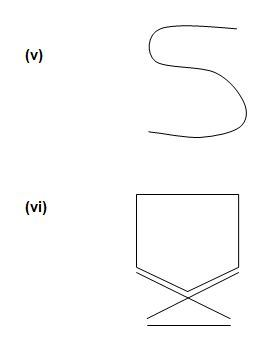Solution:

Open curve: A curve in which the beginning and end points are different or do not cut each other.

Closed curve: A curve in which the beginning and end points are the same and cut each other

By the above definitions, we can classify the given figures as follows.

(i) Open curve

(ii) Closed curve

(iii) Closed curve

(iv) Open curve

(v) Open curve

(vi) Closed curve

3. Draw a polygon and shade its interior. Also draw its diagonals, if any:

Solution:

In polygon ABCD, AC and BD are the diagonals.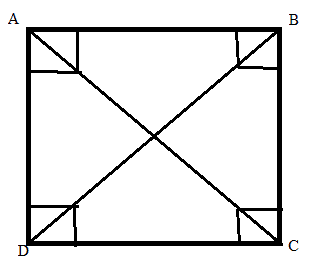4. Illustrate, if possible, each one of the following with a rough diagram:

(i) A closed curve that is not a polygon.

(ii) An open curve made up entirely of line segments.

(iii) A polygon with two sides.

Solution:

(i) Polygons are made up of straight lines, not curves.

(ii) An open curve made up entirely of line segments.

(iii) Not possible because polygons are closed figures.

5. Following are some figures: classify each of these figures on the basis of the following:

(i) Simple curve

(ii) Simple closed curve

(iii) Polygon

(iv) Convex polygon

(v) Concave polygon

(vi) Not a curveSolution:

(i) It is a simple closed curve and a concave polygon.

(ii) It is a simple closed curve and a convex polygon.

(iii) It is not a curve; hence, it is not a polygon.

(iv) It is not a curve; hence, it is not a polygon.

(v) It is a simple closed curve but not a polygon.

(vi) It is a simple closed curve but not a polygon.

(vii) It is a simple closed curve but not a polygon.

(viii) It is a simple closed curve but not a polygon.

6. How many diagonals does each of the following have?

(i) A convex quadrilateral

(ii)A regular hexagon

(iii)A triangle

Solution: An n- sided convex polygon has n(n-3)2 diagonals.

(i) A quadrilateral has 4( 4 – 3 ) 2 = 2 diagonals.

There are 2 diagonals in the convex quadrilateral.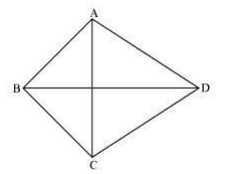(ii) A regular hexagon has 6(6-3)2 = 9 diagonals.

There are 9 diagonals in a regular hexagon.(iii) A triangle does not any diagonal in it.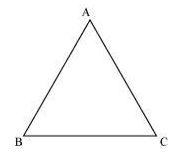7. What is a regular polygon? State the name of a regular polygon of:

(i) 3 sides (ii)4 sides (iii) 6 sides

Solution:

A polygon that has equal sides and equal angles us called a regular polygon.

(i) Equilateral triangle:(ii) Square: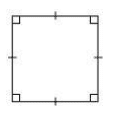(iii) Regular hexagon: